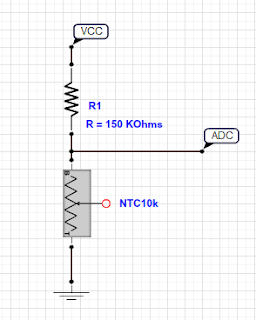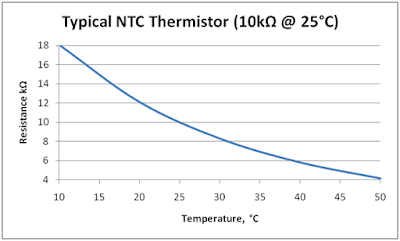## Tuesday, September 27, 2016

### Esp8266 NTC (Temperature) Thermistor Analog Reading with Arduino IDE

Hello Everyone,

In this lesson, i will show you reading temperature with an esp8266 and a ntc (thermister) sensor.

Note :
You need an ESP board having ADC pin. Only ESP8266 - 07 and ESP8266 - 12 boards have ADC pin.

I used :
-ESP8266 - 07
-10k NTC
-150k ohm resistor.

We will use very simple and basic resistor-ntc circuit. Circuit is in figure1.Figure-1

Normally, if you search on internet you will see circuits that uses 10k ntc with a 10k resistor. On my circuit i use 150k resistor. Now i will explain why i am doing it.

In figure-2, there is a graph that explaint resistance and temperature for a ntc sensor.
This sensor says NTC resistor high value is about 18-20k and 3-4k for (room temp).Figure-2
If we use 10k resistor with 10k NTC,

Assume :
NTC max resistance = 18kohm
NTC min resistance = 3k ohm
VCC = 3.3V

Here is the problem. ESP8266 ADC pin Reference is 1V.
What is that mean?
That mean is your analog voltage highest value must be 1V. So, for that reason, i will use 150k resistor and wtih 150k resistor maximum and minumum analog values are below.Theese values are good for our project. You can use a lower resistor for higher temperature resolution. In my project temperature resoulution is not very important.

ESP8266 Analog Resolution per Volts :

1V = 1024
1 / 1024 = 0.00097 V  resolution.

0.3535 V / 0.00097 V= 364
0.0647 V / 0.00097 V = 66

That means, we have 298 different temperature values. As i sad this is enough for my project.

Now we have solved hardware problem. But with this solution we have a problem with software. Functions that you can find on internet is for 10k ntc and 10k resistor and there is not enough explaniton about it.

Firstly,

We need to find instantenious resistance of NTC. You can use ESP8266 ADC pin as A0 on Arduino IDE.

int val = 0;
for(int i = 0; i < 20; i++) {
delay(1);
}

val = val / 20;
return val;
}

In my project i use filtered analog read code. This is very effective and more stable. We have read analog voltage on NTC. Now we will calculate instantenious resistance of NTC.

void loop() {
double Vcc = 3.3V;
unsigned int Rs = 150000;
double R_NTC = (Rs * V_NTC) / (Vcc - V_NTC);

Serial.println(R_NTC);
}

We have successfuly completed first step. We have calculated resistance of NTC. You can check your paramters with your multimeter but you need to now that when you touch your ntc sensor, ntc resistance will change instantly.

Second step is about calculating temperature with using instantenious resistance of ntc .

The Steinhart–Hart equation is a model of the resistance of a semiconductor at different temperatures. The equation is:

1T=A+Bln(R)+C(ln(R))3
where:
• Tis the temperature (in kelvins)
• Ris the resistance at T(in ohms)
• A, B, and C are the Steinhart–Hart coefficients which vary depending on the type and model of thermistor and the temperature range of interest. (The most general form of the applied equation contains a (ln(R))2
• term, but this is frequently neglected because it is typically much smaller than the other coefficients, and is therefore not shown above.)
Steinhart-Hart equation - Wikipedia, The Free Encyclopedia

A = 0.001129148
B = 0.000234125
C = 0.0000000876741

Now we are ready for calculate temperature.

Where:
Rs = 150000;  //150k ohm resistor
Vcc = 3.3;

double Thermister(int val) {

double V_NTC = (double)val / 1024;
double R_NTC = (Rs * V_NTC) / (Vcc - V_NTC);
R_NTC = log(R_NTC);
double Temp = 1 / (0.001129148 + (0.000234125 + (0.0000000876741 * R_NTC * R_NTC ))* R_NTC );
Temp = Temp - 273.15;
return Temp;

}
Here is the complete project code .

#include <math.h>

unsigned int Rs = 150000;
double Vcc = 3.3;
void setup() {
// put your setup code here, to run once:
Serial.begin(115200);
}

void loop() {
// put your main code here, to run repeatedly:

delay(1000);

}

int val = 0;
for(int i = 0; i < 20; i++) {
delay(1);
}

val = val / 20;
return val;
}

double Thermister(int val) {
double V_NTC = (double)val / 1024;
double R_NTC = (Rs * V_NTC) / (Vcc - V_NTC);
R_NTC = log(R_NTC);
double Temp = 1 / (0.001129148 + (0.000234125 + (0.0000000876741 * R_NTC * R_NTC ))* R_NTC );
Temp = Temp - 273.15;
return Temp;

}

You can change Rs and Vcc paramters with your own values.

Note (Important !) : If you want to use different Rs, make sure your instantenious analog input voltage value is lower than 1V.

See you soon.

1.http://esp8266tutorials.blogspot.com.tr/2016/10/arduino-esp8266-digital-smoothing.html

2.I checked my esp8266-12 (NodeMCU) and it has correct reference voltage - 3.3V

1.This tutorial not for NodeMCU or any other development boards. And thank you for interest

3.Very nice post. Thank you.

4.Hello,

Your post help me a lot. The thermistor I'm using is MF52 b=3435 10k at 25°. Any idea how to find the 3 Steinhart-Hart A,B,C values for this thermistor? I'm not sure what information I need to get these. Thank you.

5.Hello,

You can use this :

6.hi my friend i try to use this code but have a problem.
the termistore at -17,0 C have a value 73.84 C and resistor 1538

7.Hi,

I am using EPCOS B57861S103F40 Thermistor 10kΩ, 2.41 (Dia.) x 6.5mm with the adafruit feather huzzah esp8266, will it work with that program?

Thank you.

anon

1.Hello,

Yes it should work with that. You can write here if you are having problems.

2.3.I solved the problem. awesome blog thank you!!

8.Hi,
Your program works. However, I want telegram to notify me when the temperature is too high. Also, i can request for the temperature. How do i go about doing this? Hope to hear from you ASAP. Thank you.

1.Hello, i am sorry because i havent work with Telegram before.

9.Anonymous, how did you solve your problem? I'm having the same issue using the Huzzah.

1.